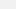# A New Number Format for Computers Could Nuke Approximation Errors for Good

## Farewell floats?

|
Apr 24 2016, 2:30pmImage: Mclek/Shutterstock

Even to the more mathematically challenged among us, it's a reasonably easy task to parse a number with a decimal point. I can give you, say, 33.333 and it doesn't take any special mental contortions for you to make sense of it (not saying that you, in particular, are mathematically challenged, but play along). So, that's 33 things and then .33 of another thing, which is almost a third of a 34th thing. Easy enough.

Computers have a funny, uneasy relationship to decimal numbers, however. Whole numbers are easy because it's easy to represent a whole number in our base-10 counting system as a binary (base-2) number. With 32 bits, or 32 digits, we can represent a huge range of whole numbers (integers or ints, in computer science), all the way up to 2147483647. Usually, that's more than enough. The problem when we start adding fractional values is that we have to have a way of encoding where exactly within a string of digits a decimal point should be located. It changes number by number.

In computing, a number with a decimal point and corresponding fractional value is represented by the floating-point data type (a float). For a 32 bit floating-point number, it turns out that we really only get 23 binary digits to represent the numerical content of a number, with the rest reserved for representing the position of the decimal point within the number (as an exponent, as in scientific notion).

The problem is less so a relatively limited range of possible values than a fundamental limitation on precision. You can see this easily enough by taking some arbitrary large number and scooting around a decimal point within it. The larger the number gets—e.g. with more digits on the left side of the decimal point—the less binary real estate we have to represent its fractional value (the right side).

John Gustafson, a computer scientist specializing high-performance computing and the namesake behind Gustafson's Law, has proposed a new solution to this seemingly unavoidable source of error (read: imprecision). He calls the new format "unum," for universal number.

The key difference is that a unum allows for the various "fields" within a binary floating-point number representation to expand and contract according to required precision. The exponential values required to scale most decimal numbers (that is, determine their decimal point locations) are usually a lot less what can be represented by five bits, as are allocated in a 32-bit float, but the current standard tries to prepare for the worst case. If the exponent field is able to shrink depending on need, that leaves more digits that can represent actual numerical content, which is good.

Here is Avogadro's number, 6.022×1023, two ways:"A unum has three additional fields that make the number self-descriptive," Gustafson explains in an interview with the ACM's Ubiquity magazine. "The 'ubit' that marks whether a number is exact or in between exact values, the size of the exponent in bits, and the size of the fraction in bits. So not only does the binary point float, the number of significant digits also floats."

There's a further approximation problem in floating-point numbers that's probably a bit harder to see, but Gustafson gives a good example.

Imagine that we have two numbers that are very close to each other: 1,000,000.7 and 1,000,000.6. Subtracting these two numbers gives us .1000000. The problem is that with .1000000 it looks an awful lot like we have six digits of precision to the right of the 1 when we really don't have any idea what's in those digits notated as zeroes. They just appeared when we did the subtraction. In the original two numbers, that precision would be off somewhere to right of the 6 and 7 digits, which is uncharted territory.

So what? The problem comes when we try to do a calculation with our new number. Like, if we add 1.0×10-12 to it we get .1000 001×10-6. Which seems right (that is, adding .1 with .000000000001 and getting .10000000001), but no. Because we never had any actual information about what was to the right of the 1 in .1. We just tricked the computer into thinking so. All we can safely do with the second, much smaller number is trash it. It's beyond our precision.

This is the utility in having a field specifying whether or not our number is exact or if it's an approximation. If the computer knows it's exact, and those extra zeroes indeed mean zero and not don't-know, than we can do the calculation. If not, we can't.

The Ubiquity interview is pretty deep, but worthwhile if you can stand more math and want to learn more about how computers (often poorly) represent information. The unum format, meanwhile, is finding some traction in the real world.

"I was just at the Supercomputing conference in Austin, TX, and I was amazed at the groundswell of interest and software efforts surrounding unum math," Gustafson said. "The Python port is already done, and several groups are working on a C port. Because the Julia language is particularly good at adding new data types without sacrificing speed, several people jumped on making a port to Julia, including the MIT founders of that language. I learned of an effort to put unum math into Java and into D, the proposed successor to C++."